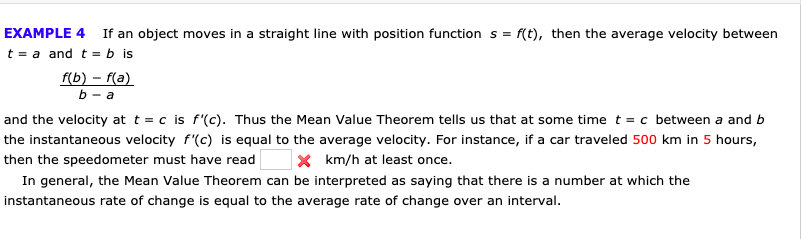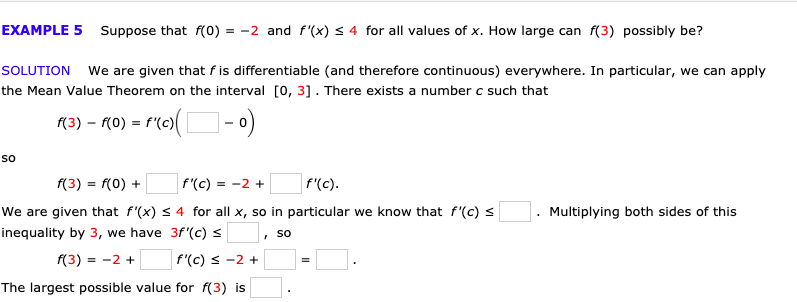# EXAMPLE 4If an object moves in a straight line with position function sf(t), then the average velocity betweent a and t = b isf(b)-f(a)b-aand the velocity at t = c is f'(c). Thus the Mean Value Theorem tells us that at some time t = c between a and bthe instantaneous velocity f'(c) is equal to the average velocity. For instance, if a car traveled 500 km in 5 hours,X km/h at least once.then the speedometer must have readIn general, the Mean Value Theorem can be interpreted as saying that there is a number at which theinstantaneous rate of change is equal to the average rate of change over an interval EXAMPLE 5Suppose that f(0) = -2 and f'(x) s 4 for all values of x. How large can f(3) possibly be?SOLUTIONWe are given that fis differentiable (and therefore continuous) everywhere. In particular, we can applythe Mean Value Theorem on the interval 0, 31. There exists a number c such thatf'(c)(3) - го)Sof'(c)f(3) f(0)f'(c)= -2We are given that f'(x) s 4 for all x, so in particular we know that f'(c) sMultiplying both sides of thisinequality by 3, we have 3f'(c)Sof(3) 2f'(c) -2The largest possible value for f(3) is

Question
76 viewshelp_outlineImage TranscriptioncloseEXAMPLE 4 If an object moves in a straight line with position function s f(t), then the average velocity between t a and t = b is f(b)-f(a) b-a and the velocity at t = c is f'(c). Thus the Mean Value Theorem tells us that at some time t = c between a and b the instantaneous velocity f'(c) is equal to the average velocity. For instance, if a car traveled 500 km in 5 hours, X km/h at least once. then the speedometer must have read In general, the Mean Value Theorem can be interpreted as saying that there is a number at which the instantaneous rate of change is equal to the average rate of change over an interval fullscreenhelp_outlineImage TranscriptioncloseEXAMPLE 5 Suppose that f(0) = -2 and f'(x) s 4 for all values of x. How large can f(3) possibly be? SOLUTION We are given that fis differentiable (and therefore continuous) everywhere. In particular, we can apply the Mean Value Theorem on the interval 0, 31. There exists a number c such that f'(c) (3) - го) So f'(c) f(3) f(0) f'(c) = -2 We are given that f'(x) s 4 for all x, so in particular we know that f'(c) s Multiplying both sides of this inequality by 3, we have 3f'(c) So f(3) 2 f'(c) -2 The largest possible value for f(3) is fullscreen
check_circle

Step 1

Hey, since there are multiple questions posted, we will answer first question. If you want any specific questi...

### Want to see the full answer?

See Solution

#### Want to see this answer and more?

Solutions are written by subject experts who are available 24/7. Questions are typically answered within 1 hour.*

See Solution
*Response times may vary by subject and question.
Tagged in

### Calculus